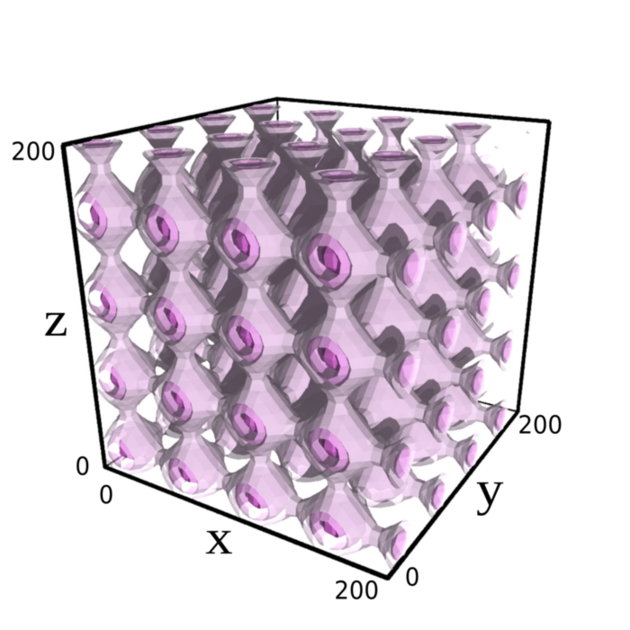Astronomical Observatory of the Jagiellonian University

# The cosmological model with a second-order perturbations

The standard cosmological model is given by the Friedmann-Lemaitre-Robertson-Walker solution. It explains many aspects of the observed Universe. However, the success of this model is accompanied by some mysteries and problems to be solved. The model predicts the existence of unknown, hypothetical forms of matter like dark matter and dark energy.Illustration: Exemplary isodensity surfaces of the considered cosmological model. Credit: The Authors.

Moreover, there appears a discrepancy between the local observations of the Lemaitre-Hubble constant value and its value deduced from the cosmic microwave background modelling. FLRW model is spatially homogeneous. The matter density is equal in every position in space. One of the attempts to understand the above issues is a consideration of inhomogeneous cosmological models. The work done in Department of Relativistic Astrophysics and Cosmology gives the inhomogeneous cosmological model constructed within the second-order perturbation theory.

The perturbation theory deals with a small corrections to the background solution, which in our case is the FLRW model. In cosmology, the linear perturbation theory is well understood. In this framework, one should leave in the equations the terms proportional to the perturbation but neglect the quadratic and higher-order terms. Unfortunately, the current-day inhomogeneities cannot be modelled satisfactorily by the linear perturbation theory. The correction under consideration should be small enough to fulfil the criteria of the linear perturbation theory, while the difference between the density at a galaxy cluster centre and the mean density of the universe is too big. The higher-order perturbations are then justified.

We construct every cosmological solution within the framework of General Relativity, which is our best theory of gravitation. Its fundamental components are the Einstein equations — the set of complicated non-linear partial differential equations. Because a complexity of these equations, it is not easy to find a solution to the cosmological perturbation theory in the order higher than linear. In the presented article, the author simplifies Einstein equations by imposing specific symmetry conditions. In this way, the second-order solution to the cosmological perturbation theory is constructed.

This work generalizes the earlier results [Maj 2019], where the background spacetime was spatially flat. The spatial flatness of the background is a common simplification in the perturbation theory. The actual paper is not restricted to the spatially flat background. Any FLRW model filled with dust matter and possibly non-zero cosmological constant can be the taken as the background spacetime, even for a non-zero spatial curvature. The most general FLRW model perturbed up to the second order in the presented way gives us a possibility to answer interesting question: how the local structures, modelled by these perturbations, affect cosmological observables?

Original publication: S. Sikora, Second-order dust perturbations of the non-flat FLRW model with the positive cosmological constant, Classical and Quantum Gravity 40, 025002, (2023).

The research was conducted at the Department of Relativistic Astrophysics and Cosmology of the Jagiellonian University’s Astronomical Observatory (OA UJ).

Contact:
 Szymon Sikora Astronomical Observatory Jagiellonian University S.Sikora [@] oa.uj.edu.pl